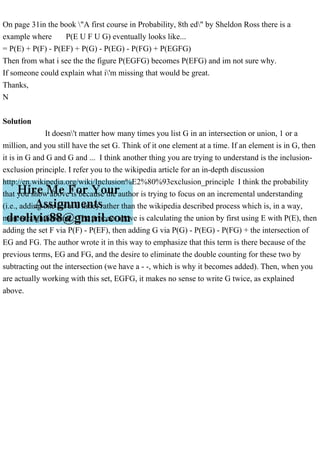Publicité

# On page 31in the book A first course in Probability, 8th ed by S.pdf

25 Mar 2023Prochain SlideShareOn page 14 of The Call Of The Wild, Jack London writes, Invague .pdf
Chargement dans ... 3
1 sur 1
Publicité

### On page 31in the book A first course in Probability, 8th ed by S.pdf

1. On page 31in the book "A first course in Probability, 8th ed" by Sheldon Ross there is a example where P(E U F U G) eventually looks like... = P(E) + P(F) - P(EF) + P(G) - P(EG) - P(FG) + P(EGFG) Then from what i see the the figure P(EGFG) becomes P(EFG) and im not sure why. If someone could explain what i'm missing that would be great. Thanks, N Solution It doesn't matter how many times you list G in an intersection or union, 1 or a million, and you still have the set G. Think of it one element at a time. If an element is in G, then it is in G and G and G and ... I think another thing you are trying to understand is the inclusion- exclusion principle. I refer you to the wikipedia article for an in-depth discussion http://en.wikipedia.org/wiki/Inclusion%E2%80%93exclusion_principle I think the probability that you show above is because the author is trying to focus on an incremental understanding (i.e., adding one set at a time) rather than the wikipedia described process which is, in a way, more straightforward. The process above is calculating the union by first using E with P(E), then adding the set F via P(F) - P(EF), then adding G via P(G) - P(EG) - P(FG) + the intersection of EG and FG. The author wrote it in this way to emphasize that this term is there because of the previous terms, EG and FG, and the desire to eliminate the double counting for these two by subtracting out the intersection (we have a - -, which is why it becomes added). Then, when you are actually working with this set, EGFG, it makes no sense to write G twice, as explained above.
Publicité